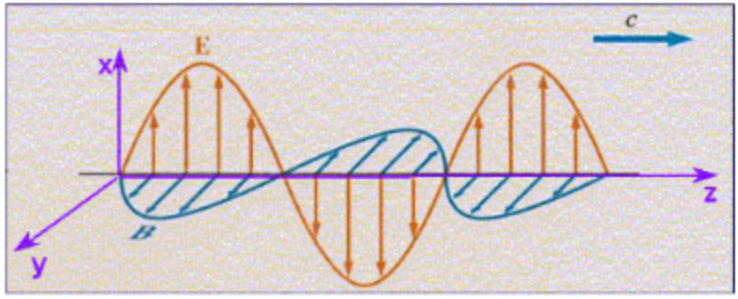# Problem: 1)  Which equation correctly describes the electromagnetic wave shown above? Briefly explain your reasoning.a) Ex  = Eo sin(kz + wt)b) Ey = Eo sin(kz - at)c) By = Bo sin(kz - wt) 2) Which of the following actions will increase the energy carried by this wave? Briefly explain your reasoning.a) Increase E keeping w constantb) Increase w keeping E constantc) Both of the above actions will increase the energy of the waved) Neither of the above actions will increase the energy of the wave.

###### FREE Expert Solution

For an electromagnetic wave,

Electric field:

$\overline{)\stackrel{\mathbf{⇀}}{{\mathbf{E}}_{\mathbf{x}}}{\mathbf{=}}{{\mathbf{E}}}_{{\mathbf{0}}}{\mathbf{s}}{\mathbf{i}}{\mathbf{n}}{\mathbf{\left(}}{\mathbf{kz}}{\mathbf{-}}{\mathbf{\omega }}{\mathbf{t}}{\mathbf{\right)}}}$

100% (308 ratings)###### Problem Details

1)Which equation correctly describes the electromagnetic wave shown above? Briefly explain your reasoning.

a) E = Eo sin(kz + wt)

b) E= Eo sin(kz - at)

c) B= Bo sin(kz - wt)

2) Which of the following actions will increase the energy carried by this wave? Briefly explain your reasoning.

a) Increase E keeping w constant

b) Increase w keeping E constant

c) Both of the above actions will increase the energy of the wave

d) Neither of the above actions will increase the energy of the wave.